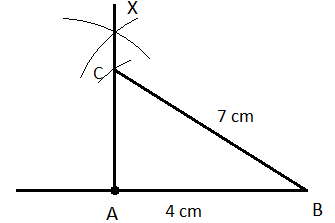Courses
Courses for Kids
Free study material
Offline Centres
More

# Practical Geometry Class 7 Notes CBSE Maths Chapter 10 [Free PDF Download]Last updated date: 27th Nov 2023
Total views: 591.3k
Views today: 10.91k

## Revision Notes for CBSE Class 7 Maths Chapter 10 - Free PDF Download

Free PDF download of Class 7 Maths Chapter 10 - Practical Geometry Revision Notes & Short Key-notes prepared by expert Maths teachers from latest edition of CBSE(NCERT) books. To register Maths Tuitions on Vedantu.com to clear your doubts.

Also, check CBSE Class 7 Maths revision notes for All chapters:

## Access Class 7 Maths Chapter 10 - Practical Geometry Notes

• Construction of a Line parallel to a given line, through a point not on the line:

1. Construct a line $l$ with the help of a ruler and mark a point $\text{A}$, but not on the line. Join that point with another point $\text{B}$ which is on the line.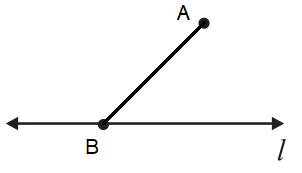1. Now, placing the compass needle at $\text{B}$, draw an arc cutting the line $l$ and $\overleftrightarrow{\text{AB}}$ at points $\text{C}$ and $\text{D}$ respectively.  Now, similarly, placing the compass needle at $\text{A}$, without changing the radius, draw another arc $\overset\frown{\text{EF}}$ cutting the $\overleftrightarrow{\text{AB}}$ at $\text{G}$.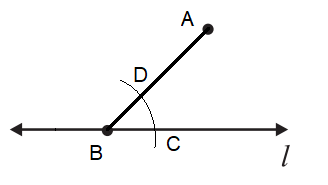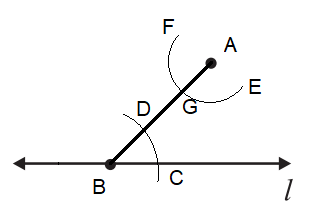1. Now, place the needle of the compass at $\text{C}$, and measure $\text{CD}$ as the radius, then put the needle at $\text{G}$, draw an arc cutting $\overset\frown{\text{EF}}$ at $\text{H}$.

2. Finally, draw a line $m$ joining $\overleftrightarrow{\text{AH}}$.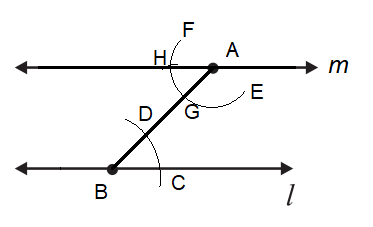• Properties of a triangle:

1. A triangle's exterior angle is equal to the sum of its inner opposite angles.

2. The sum of a triangle's three angles is $180{}^\circ$.

3. The sum of the lengths of any two triangle sides is greater than the third side's length.

4. The square of the hypotenuse length is equal to the sum of the squares of the other two sides in any right-angled triangle.

• Constructing a Triangle when the lengths of its three sides are known (SSS Criterion):

1. Draw a line segment $\overline{\text{AB}}\text{ = 4 cm}$ with the help of a ruler and pencil, then place the compass needle at point $\text{A}$, taking the desired length as radius, say 5 cm, draw an arc $\overset\frown{\text{KL}}$.

2. Similarly, placing the compass needle at $\text{B}$, draw another arc $\overset\frown{\text{XY}}$ cutting $\overset\frown{\text{KL}}$, taking the desired radius, say 6 cm (the length of the third side of the required triangle).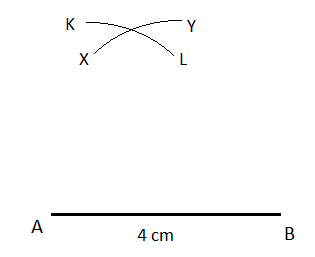1. Mark the intersection of both the arcs as point $\text{C}$, now by joining $\overline{\text{AC}}$ and $\overline{\text{BC}}$, we will get the triangle.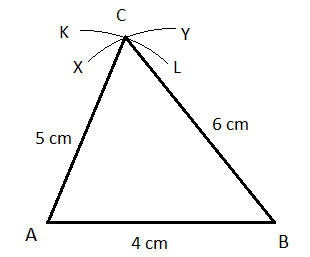• Constructing a Triangle when the lengths of two sides and the measure of the angle between them are known (SAS Criterion):

1. Draw a line segment $\overline{\text{AB}}\text{ = 4 cm}$ with the help of a ruler and pencil, then draw another line from point $\text{A}$, making the desired angle, say $60{}^\circ$.

2. Now, taking $\text{A}$ as centre, placing the compass needle there, draw an arc $\overset\frown{\text{KL}}$, with the desired radius (the given length of the other side) cutting $\overrightarrow{\text{AX}}$ at $\text{C}$.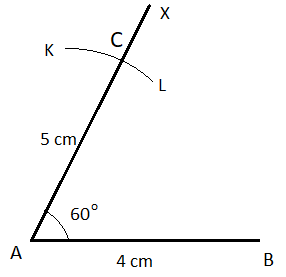1. Finally, join $\overline{\text{BC}}$ to get the required triangle.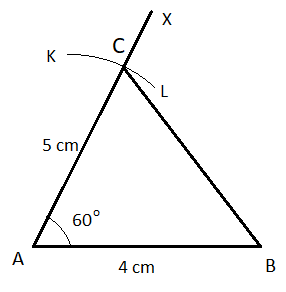• Constructing a Triangle when the measures of two angles and the length of the side included between them are known (ASA Criterion):

1. Draw a line segment $\overline{\text{AB}}\text{ = 4 cm}$ with the help of a ruler and pencil, then draw another line from point $\text{A}$, making the desired angle, say $30{}^\circ$.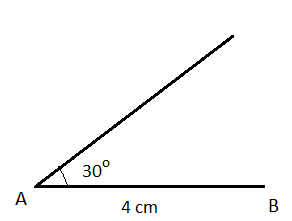1. Draw another angle, say $75{}^\circ$ at $\text{B}$ and extend the line to meet the angle subtended from point $\text{A}$, naming it point $\text{C}$.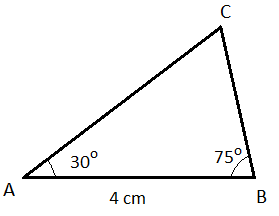• Constructing a Right-angled triangle when the length of one side and its hypotenuse are known (RHS Criterion):

1. Draw a line segment $\overline{\text{AB}}\text{ = 4 cm}$ with the help of a ruler and pencil, then draw another line from point $\text{A}$, making the desired right angle.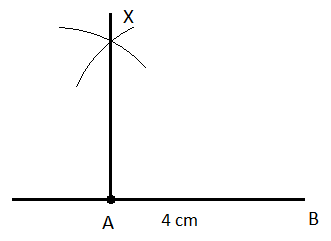1. Now, placing the needle of the compass at $\text{B}$ and taking the measure of another side (hypotenuse), say 7 cm, draw an arc cutting the line $\overline{\text{AX}}$  at $\text{C}$ .

2. Joining the $\text{B}$ with the point of intersection of this arc at $\text{C}$, will give us the required triangle.